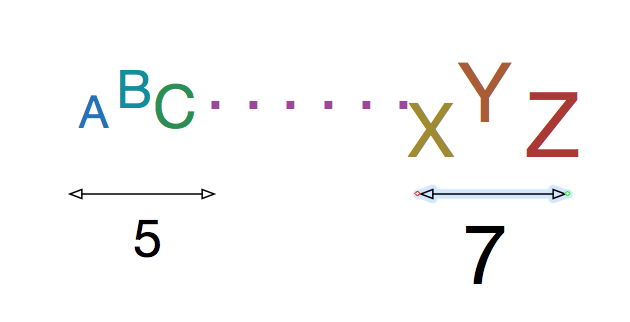# As Simple as 3-5-7The sum of $N$ real numbers (not necessarily unique) is 20. The sum of the 3 smallest of these numbers is 5. The sum of the 3 largest is 7. Which of the following are possible values for $N$?

I. 9
II. 10
III. 11

×